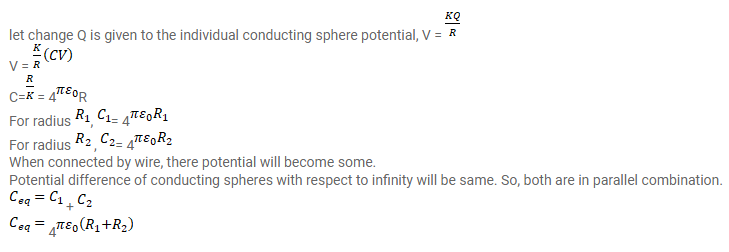# Two conducting spheres of radii R1and R2Question:

Two conducting spheres of radii $\mathrm{R}_{1}$ and $\mathrm{R}_{2}$ are kept widely separated from each other. What are their individual capacitance? If the spheres are connected by a metal wire, what will be the capacitance of the combination? Think in terms of series parallel connections.

Solution: